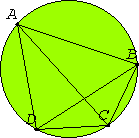# Proposition 22

The sum of the opposite angles of quadrilaterals in circles equals two right angles.

Let ABCD be a circle, and let ABCD be a quadrilateral in it.

I say that the sum of the opposite angles equals two right angles.Join AC and BD.

I.32

Then, since in any triangle the sum of the three angles equals two right angles, the sum of the three angles CAB, ABC, and BCA of the triangle ABC equals two right angles.

III.21

But the angle CAB equals the angle BDC, for they are in the same segment BADC, and the angle ACB equals the angle ADB, for they are in the same segment ADCB, therefore the whole angle ADC equals the sum of the angles BAC and ACB.

Add the angle ABC to each. Therefore the sum of the angles ABC, BAC, and ACB equals the sum of the angles ABC and ADC. But the sum of the angles ABC, BAC, and ACB equals two right angles, therefore the sum of the angles ABC and ADC also equal two right angles.

Similarly we can prove that the sum of the angles BAD and DCB also equals two right angles.

Therefore the sum of the opposite angles of quadrilaterals in circles equals two right angles.

Q.E.D.

## Guide

This proposition is used in III.31 and III.32.